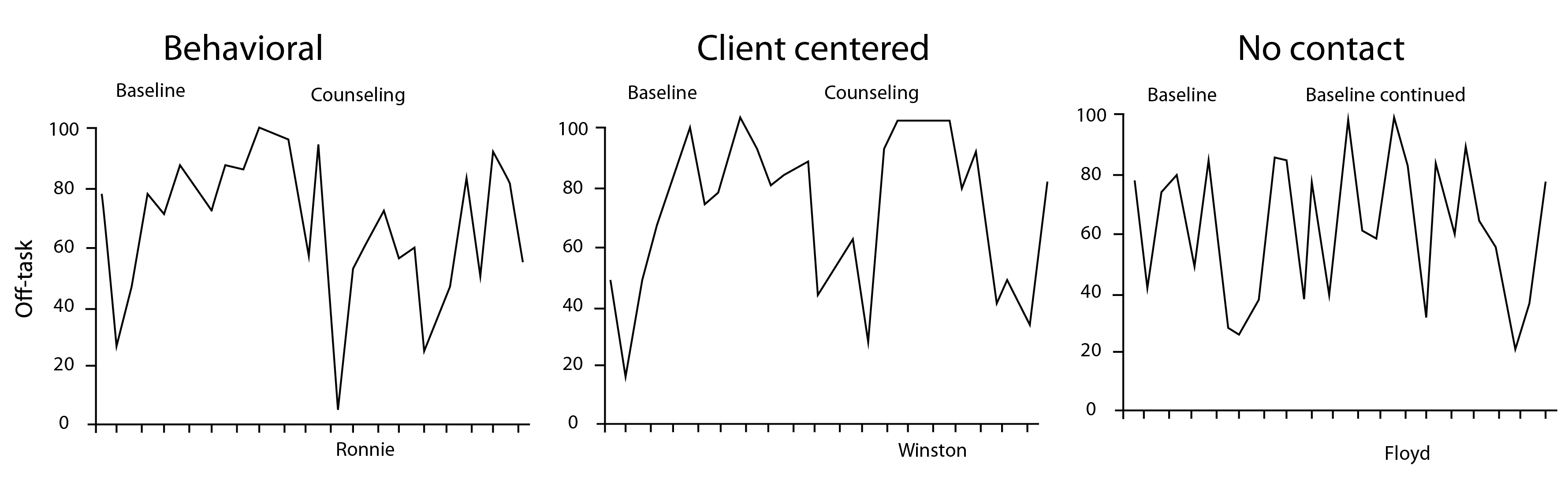Course Content

# Time Series Analysis

1. Time Series: Let's Start

2. Time Series Processing

3. Time Series Visualization

Time Series Analysis

##Predict the Emergence of Repeat Customers

How is the problem of predicting customer behavior different from, for example, the problem of predicting the total profit of a supermarket? The answer is simple - it's data.In order to predict customer behavior, you need to "build" predictive models for each of the customers while taking into account the most common behavior patterns of all customers. What does this mean for us?

The fact is that usual ARIMA model may be ineffective in this task. Currently, neural network models such as LSTM and Transformers are the most widely used.

Neural network models are trained on all our multidimensional data and build an "equation" (modifies the model parameters), which further predicts incoming data.